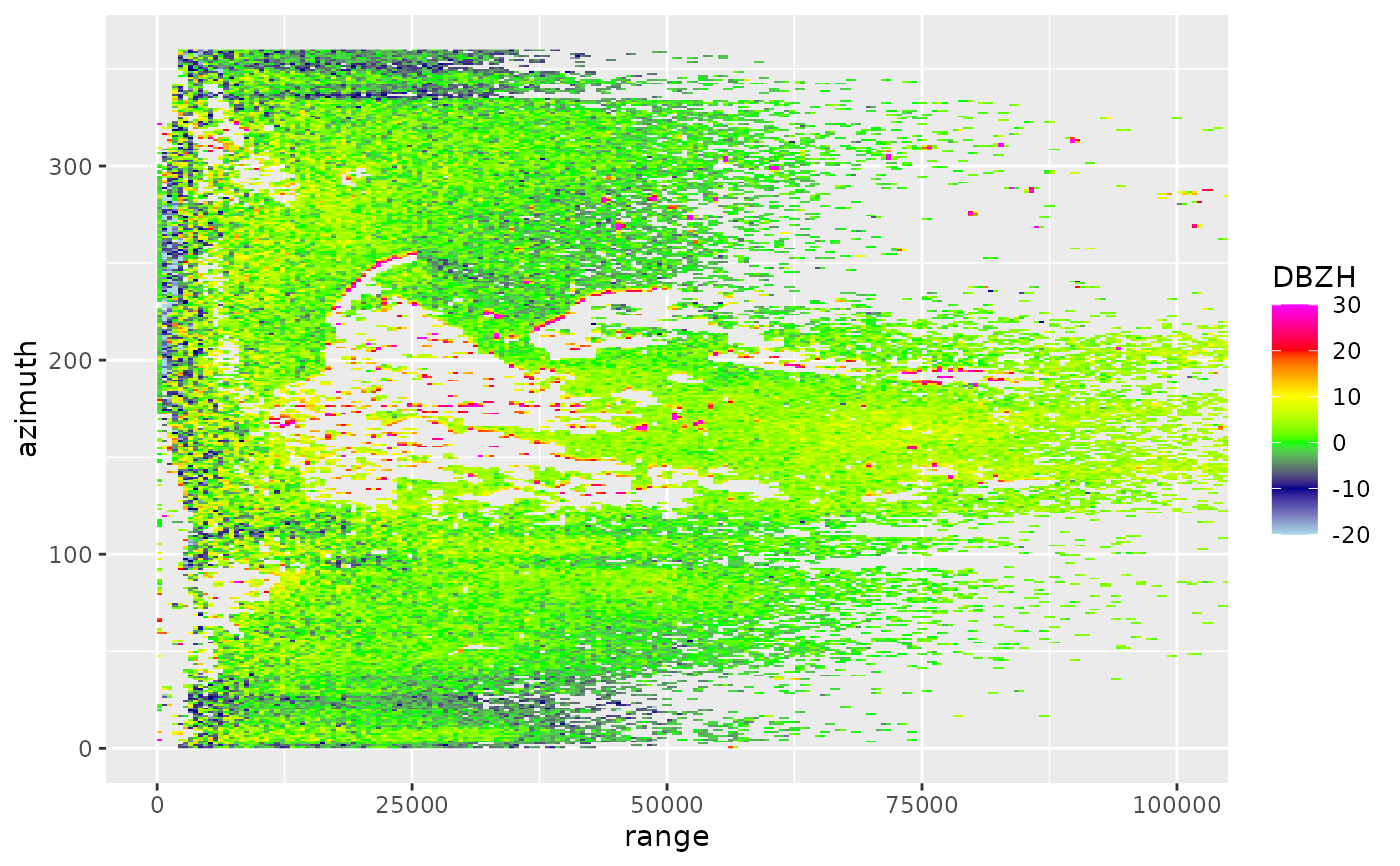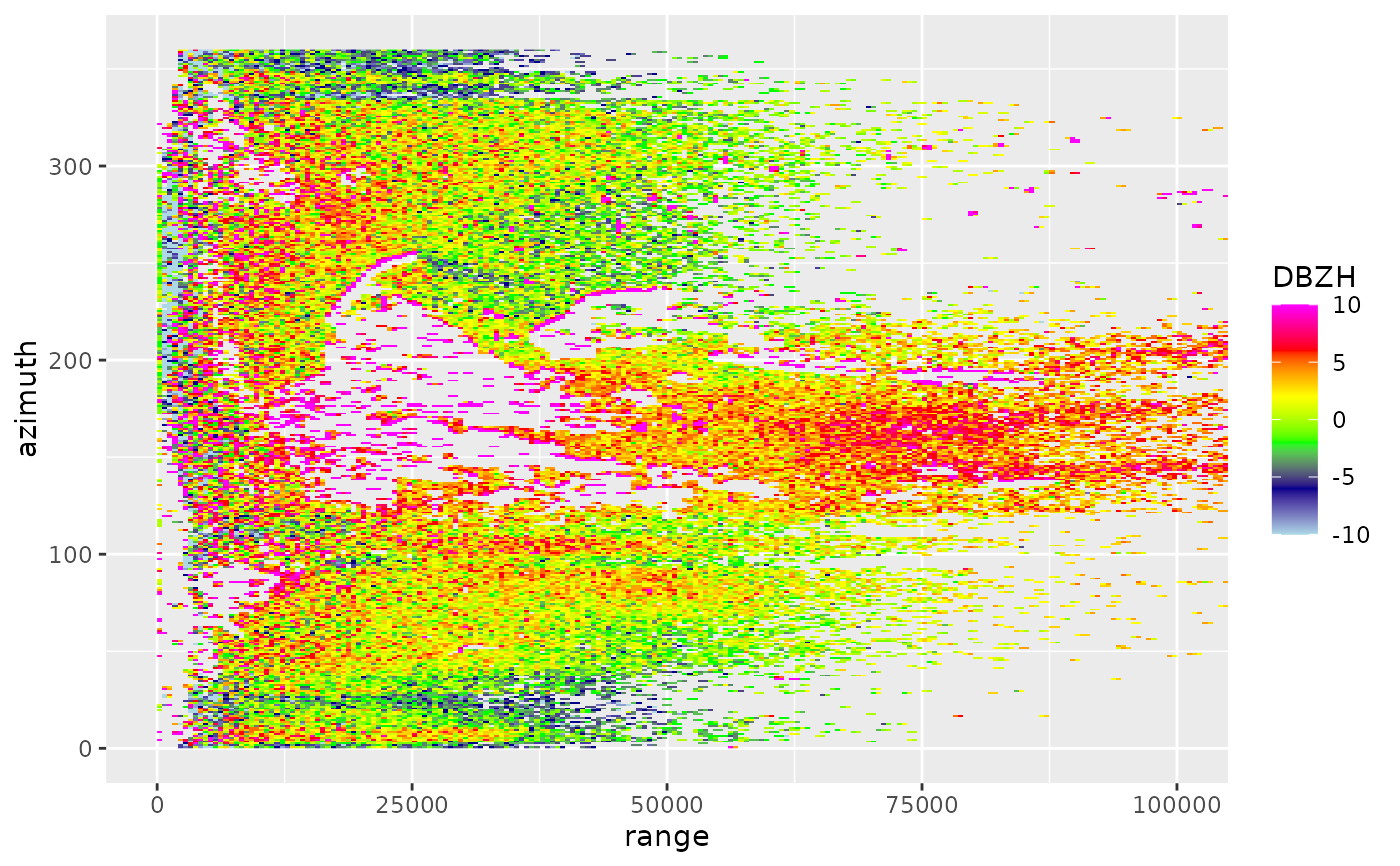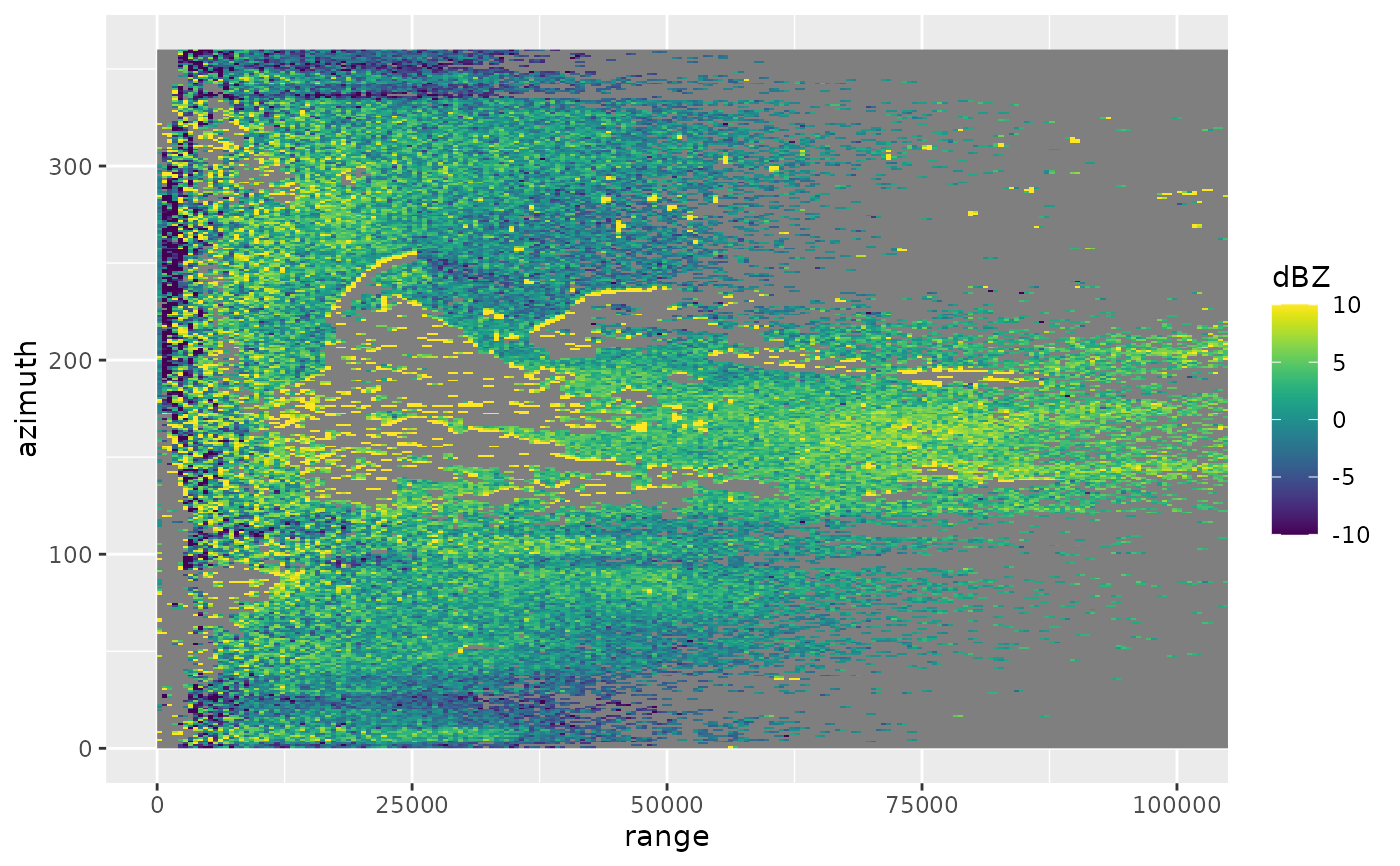Plots a scan in polar coordinates. For plots in Cartesian coordinates, see project_to_ppi

# S3 method for scan
plot(
x,
param,
xlim = c(0, 1e+05),
ylim = c(0, 360),
zlim = c(-20, 20),
na.value = "transparent",
...
)

## Arguments

x

An object of class scan.

param

The scan parameter to plot, see details below.

xlim

Range of x (range, distance from radar) values to plot.

ylim

Range of y (azimuth) values to plot.

zlim

The range of parameter values to plot.

na.value

ggplot argument setting the plot color of NA values

...

Arguments passed to low level ggplot function.

## Details

Available scan parameters for plotting can by printed to screen by summary(x). Commonly available parameters are:

"DBZH", "DBZ"

(Logged) reflectivity factor (dBZ)

"TH", "T"

(Logged) uncorrected reflectivity factor (dBZ)

"VRADH", "VRAD"

"RHOHV"

Correlation coefficient (unitless). Correlation between vertically polarized and horizontally polarized reflectivity factor

"PHIDP"

Differential phase (degrees)

"ZDR"

(Logged) differential reflectivity (dB)

The scan parameters are named according to the OPERA data information model (ODIM), see Table 16 in the ODIM specification.

## Examples

# load an example scan:
data(example_scan)

# print to screen the available scan parameters
summary(example_scan)
#>                   Polar scan (class scan)
#>
#>      parameters:  DBZH VRADH RHOHV ZDR PHIDP
#> elevation angle:  0.5 deg
#>            dims:  480 bins x 360 rays

# make ppi for the scan
# plot the reflectivity param:
plot(example_scan, param = "DBZH")# \dontrun{
# change the range of reflectivities to plot, from -10 to 10 dBZ:
plot(example_scan, param = "DBZH", zlim = c(-10, 10))# change the scale name and colour scheme, using viridis colors:
plot(example_scan, param = "DBZH", zlim = c(-10, 10)) + viridis::scale_fill_viridis(name = "dBZ")
#> Scale for 'fill' is already present. Adding another scale for 'fill', which
#> will replace the existing scale.# }